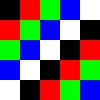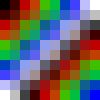# Indexed image in 5 minutesThis demonstration shows you how to work with indexed image using IndirectArrays.jl

An indexed image consists of two parts: the indices and the palatte. The palatte keeps track of all possible pixel values of an image, while the indices consists of the palatte index of each pixel.

using Images

The following is a normally used representation of image, i.e., an image as an array of Colorants.

img = [
RGB(0.0, 0.0, 0.0) RGB(1.0, 0.0, 0.0) RGB(0.0, 1.0, 0.0) RGB(0.0, 0.0, 1.0) RGB(1.0, 1.0, 1.0)
RGB(1.0, 0.0, 0.0) RGB(0.0, 1.0, 0.0) RGB(0.0, 0.0, 1.0) RGB(1.0, 1.0, 1.0) RGB(0.0, 0.0, 0.0)
RGB(0.0, 1.0, 0.0) RGB(0.0, 0.0, 1.0) RGB(1.0, 1.0, 1.0) RGB(0.0, 0.0, 0.0) RGB(1.0, 0.0, 0.0)
RGB(0.0, 0.0, 1.0) RGB(1.0, 1.0, 1.0) RGB(0.0, 0.0, 0.0) RGB(1.0, 0.0, 0.0) RGB(0.0, 1.0, 0.0)
RGB(1.0, 1.0, 1.0) RGB(0.0, 0.0, 0.0) RGB(1.0, 0.0, 0.0) RGB(0.0, 1.0, 0.0) RGB(0.0, 0.0, 1.0)]Alternatively, we could save it in the indexed image format:

indices = [1 2 3 4 5; 2 3 4 5 1; 3 4 5 1 2; 4 5 1 2 3; 5 1 2 3 4] # i records the pixel value palatte[i]
palatte = [RGB(0.0, 0.0, 0.0), RGB(1.0, 0.0, 0.0), RGB(0.0, 1.0, 0.0), RGB(0.0, 0.0, 1.0), RGB(1.0, 1.0, 1.0)]

palatte[indices] == img
true

This is doable because it follows the Julia indexing rules that indices is an array of scalar indices, and the dimensionality of the output is the dimensionality of the indices. For example, the following equivalence holds:

palatte[[1, 2]] == [palatte, palatte]
palatte[[1 2; 2 1]] == [palatte palatte; palatte palatte]

Now, let's analyze the resource usage of these two approaches. For this example, we are storing the data using type Float64, where each Float64 number requires 8 bytes (1 byte=8bits). Hence the normal representation format requires 5*5*3*8 = 600 bytes storage in total. As a comparison, we only need 5*5*8 + 5*3*8 = 320 bytes storage if we use the indexed image format. This is why images with few distinct values can often be encoded more compactly as an indexed image.

Although it does compress the data in this example, in real world applications, it is not always clear whether you should or should not use indexed image format. There are two main drawbacks of it:

• indexed images can require more memory if length(unique(img)) is too large.
• indexing into an indexed image requires two getindex operations, so using it can be slower than a direct image representation (and not amenable to SIMD vectorization). This is a typical space-time tradeoff case.

Benchmarks are always recommended before you choose to use the indexed image format.

Using indexed image format with two seperate arrays can be inconvinient, hence IndirectArrays.jl provides an array abstraction to union these two data:

using IndirectArrays

indexed_img = IndirectArray(indices, palatte)
img == indexed_img
true

Under the hook, it is just a simple struct that subtypes AbstractArray:

# no need to run this
struct IndirectArray{T,N,A,V} <: AbstractArray{T,N}
index::A
values::V
end
indexed_img.index === indices, indexed_img.values === palatte
(true, true)

Since IndirectArray is just an array, common image operations are applicable to this type, for example:

imresize(indexed_img; ratio=2) # no longer an IndirectArray